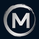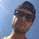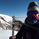87288浏览
This is a simple RSI based signal indicator. It is intended for algorithmic trading by bots, currently working one up for http://bitforex.uk.to and http://okcoin.uk.to to use this.

For the best results leave it on 1-Hour time-frame. It also works best on bitcoin and stocks, not so much oil . GBP/USD and AUD/USD it fairs well too.

When RSI touches 70 it gives a Buy signal and when RSI touches 30 it gives a sell signal. The position is then held out until an opposite signal has been given. If the back-testing shows poor results but looks as though the inverse would be good, there is an easy toggle in the settings to flip it. Also in the code is a nifty method to pragmatically keep a variable's value from resetting each candle until later changed.

MSFT Stock:

```study("RSI Algo", overlay=true)
myPeriod = input(defval=14, type=integer, title="Period")
myThresholdUp = input(defval=70, type=float, title="Upper Threshold")
myThresholdDn = input(defval=30, type=float, title="Lower Threshold")
myAlgoFlipToggle = input(defval=false, type=bool, title="Imverse Algorthim")
myLineToggle = input(defval=true, type=bool, title="Show Lines")
myLabelToggle = input(defval=true, type=bool, title="Show Labels")
myRSI=rsi(close, myPeriod)
buy = myAlgoFlipToggle ? falling(myRSI,1) and cross(myRSI, myThresholdDn) : rising(myRSI, 1) and cross(myRSI,myThresholdUp)
sell = myAlgoFlipToggle ? rising(myRSI, 1) and cross(myRSI,myThresholdUp) : falling(myRSI,1) and cross(myRSI, myThresholdDn)
myPosition = buy==1 ? 0 : sell==1 or myPosition==1 ? 1 : 0
trendColor = buy ? red : sell ? green : na
plot(myLineToggle ? buy and myPosition==1 ? low - 0.004: sell and myPosition==0 ? high + 0.004 : na : na, color=trendColor, style=line, linewidth=4, editable=false)
plotshape(myLabelToggle ? buy and myPosition==1 ? low - 0.005 : na : na, style=shape.labelup, location=location.absolute, text="Buy", transp=0, textcolor = white, color=black, editable=false)
plotshape(myLabelToggle ? sell and myPosition==0 ? high + 0.005 : na : na, style=shape.labeldown, location=location.absolute, text="Sell", transp=0, textcolor = white, color=black, editable=false)```

## 评论